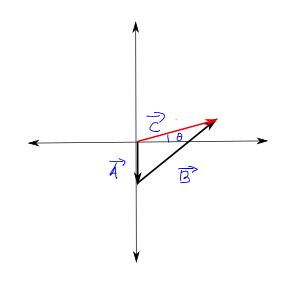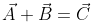×
Get Full Access to University Physics - 13 Edition - Chapter 1 - Problem 28e
Get Full Access to University Physics - 13 Edition - Chapter 1 - Problem 28e

×

# For the vectors and in Fig. E1.24, use a scale drawing toISBN: 9780321675460 31

## Solution for problem 28E Chapter 1

University Physics | 13th Edition

• Textbook Solutions
• 2901 Step-by-step solutions solved by professors and subject experts
• Get 24/7 help from StudySoup virtual teaching assistantsUniversity Physics | 13th Edition

4 5 1 395 Reviews
20
4
Problem 28E

For the vectors and in Fig. E1.24, use a scale drawing to find the magnitude and direction of (a) the vector sum and (b) the vector difference Use your answers to find the magnitude and direction of (c) and (d) (See also Exercise 1.31 for a different approach.)

Step-by-Step Solution:

Problem 28E

For the vectors and in Fig. E1.24, use a scale drawing to find the magnitude and direction of (a) the vector sum A+B and (b) the vector difference A-B Use your answers to find the magnitude and direction of (c) -A-Band (d) B-A(See also Exercise 1.31 for a different approach.)

Step By Step Solution

Step 1 of 4

(a)

Drawing the vectors to scale:

Scale:2m=1cmMeasuring the magnitude and direction of vector C,

Magnitude of vector C = 9m

Direction of Vector C = 34 degrees from positive x axis.

Step 2 of 4

Step 3 of 4

##### ISBN: 9780321675460

Unlock Textbook Solution